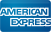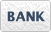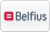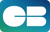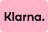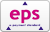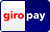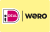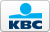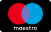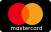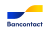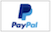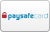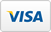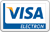EUR

# Pattern bit / Top bearing straight bits

Titman
Guide and trim
Guide and trim
€49,50 €49,50

Titman
Pattern bit Z=2 D=19 L=12 S=8
Pattern bit Z=2 D=19 L=12 S=8
€109,78 €109,78 €99,00 €99,00

Titman
Pattern bit Z=2 D=19 L=20 S=8
Pattern bit Z=2 D=19 L=20 S=8
€109,78 €109,78 €99,00 €99,00

Titman
Straight bit Z=2 D=19 L=20 S=12
Straight bit Z=2 D=19 L=20 S=12
€114,00 €114,00 €105,00 €105,00

Titman
Straight bit Z=2 D=19 L=30 S=12
Straight bit Z=2 D=19 L=30 S=12
€105,00 €105,00

Titman
Straight bit Z=2 D=19 L=30 S=8
Straight bit Z=2 D=19 L=30 S=8 mm
€128,37 €128,37 €115,53 €115,53

Titman
Pattern bit with replaceable knives L50/4 Z=2 D=19 L=50 S=12
Pattern bit with replaceable knives L50/4 Z=2 D=19 L=50 S=12
€133,26 €133,26 €127,00 €127,00

Titman
Pattern bit with replaceable knivesL60/4 Z=2 D=19 L=60 S=12
Pattern bit with replaceable knives L60/4 Z=2 D=19 L=60 S=12
€164,15 €164,15 €155,00 €155,00

Showing 1 - 12 of 12
##### Cutter